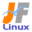Linux Kernel 2.4 Documentation: /usr/src/linux/Documentation/smp.txt

smp.txt

Linux \$B\$G(B SMP \$B\$r;H\$&J}K!(B [\$B%W%l%\$%s%F%-%9%HHG(B]
• \$B86Cx:n
• \$BK]Lu
• \$B%P!<%8%g%s(B: 2.4.0
• \$BK]LuF|;~(B: 1999/04/13
```

To set up SMP

Configure the kernel and answer Y to CONFIG_SMP.

SMP \$B\$r;H\$(\$k\$h\$&\$K\$9\$k\$K\$O!"%+!<%M%k\$r(B config \$B\$7(B CONFIG_SMP \$B\$K(B Y \$B\$r(B
\$B@_Dj\$7\$F\$/\$@\$5\$\$!#(B

If you are using LILO, it is handy to have both SMP and non-SMP
kernel images on hand. Edit /etc/lilo.conf to create an entry
for another kernel image called "linux-smp" or something.

LILO \$B\$r;H\$C\$F\$\$\$k>l9g!"(BSMP \$B\$H(B \$BHs(B SMP \$B\$N%+!<%M%k%\$%a!<%8\$NN>J}\$rCV\$\$\$F\$*(B
\$B\$/\$HJXMx\$G\$9!#(B/etc/lilo.conf \$B\$rJT=8\$7\$F!"(B"linux-smp" \$B\$N\$h\$&\$JL>A0\$r(B
\$B\$D\$1\$?\$b\$&0l\$D\$N!JLuCm!'\$D\$^\$j(B SMP \$BBP1~\$N!K%+!<%M%k%\$%a!<%8\$X\$N%(%s%H%j!<(B
\$B\$r:n@.\$7\$F\$/\$@\$5\$\$!#(B

The next time you compile the kernel, when running a SMP kernel,
edit linux/Makefile and change "MAKE=make" to "MAKE=make -jN"
(where N = number of CPU + 1, or if you have tons of memory/swap
you can just use "-j" without a number). Feel free to experiment
with this one.

\$Bl9g!"(B
linux/Makefile \$B\$rJT=8\$7\$F(B "MAKE=make" \$B\$r(B "MAKE=make -jN"\$B!J(BN \$B\$O(B CPU \$B?t(B +1
\$B\$G\$9\$,!"\$?\$/\$5\$s\$N%a%b%j!?%9%o%C%W\$,\$"\$k>l9g\$O?t;zL5\$7\$N(B"-j"\$B\$@\$1\$K\$9\$k\$3(B
\$B\$H\$b\$G\$-\$^\$9!K\$K\$7\$F\$/\$@\$5\$\$!#5\$3Z\$K\$\$\$-\$^\$7\$g\$&!#(B

Of course you should time how long each build takes :-)
Example:
make config
time -v sh -c 'make dep ; make clean install modules modules_install'

\$B\$b\$A\$m\$s%S%k%I\$9\$k\$N\$K\$I\$l\$0\$i\$\$\$N;~4V\$,\$+\$+\$C\$?\$N\$+\$rB,Dj\$7\$?J}\$,(B
\$B\$h\$\$\$G\$7\$g\$&(B :-)
\$BNc!'(B
make config
time -v sh -c 'make dep ; make clean install modules modules_install'

If you are using some Compaq MP compliant machines you will need to set
the operating system in the BIOS settings to "Unixware" - don't ask me
why Compaqs don't work otherwise.

Compaq MP \$B=`5r\$N%^%7%s\$r;HMQ\$7\$F\$\$\$k>l9g!"(BBIOS \$B\$K\$"\$k(B OS \$B\$N@_Dj\$r(B
"Unixware" \$B\$K\$9\$kI,MW\$,\$"\$k\$G\$7\$g\$&(B \$B!](B Compaq \$B\$N%^%7%s\$OB>\$N@_Dj\$@\$H\$J\$<(B
\$BF0:n\$7\$J\$\$\$+\$r;d\$KJ9\$+\$J\$\$\$G\$/\$@\$5\$\$\$M!#(B
---------------
\$BF|K\8lLu!'Aa@n(B \$B?N(B (1999/04/13)
```